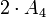Supergroups of alternating group:A4

This article gives specific information, namely, supergroups, about a particular group, namely: alternating group:A4.
View supergroups of particular groups | View other specific information about alternating group:A4

This article discusses some of the groups that admit the alternating group of degree four as a subgroup, quotient group, or subquotient.

Note that unlike the discussion of the subgroup structure of alternating group:A4, this discussion is necessarily not comprehensive, because there are infinitely many groups containing the alternating group of degree four.

Subgroups and quotients: essential minimalist examples

Subgroups: making all the automorphisms inner

Further information: symmetric group:S4, A4 in S4

The outer automorphism group of alternating group:A4 is cyclic group:Z2 and the automorphism group is symmetric group:S4. Since$A_4$ is centerless, it equals its inner automorphism group and hence embeds as a subgroup of index two inside symmetric group:S4.

In particular, symmetric group:S4 is the unique group containing alternating group:A4 as a NSCFN-subgroup (a normal fully normalized subgroup that is also a self-centralizing subgroup).

Quotients: Schur covering groups

Further information: special linear group:SL(2,3), center of special linear group:SL(2,3)

The Schur multiplier of alternating group:A4 is cyclic group:Z2. There is a unique corresponding Schur covering group, namely the group special linear group:SL(2,3), where the center of special linear group:SL(2,3) is isomorphic to the Schur multiplier cyclic group:Z2 and the quotient is alternating group:A4.

The Schur covering group$SL(2,3)$ is also denoted$2 \cdot A_4$ to indicate that it is a double cover of alternating group.